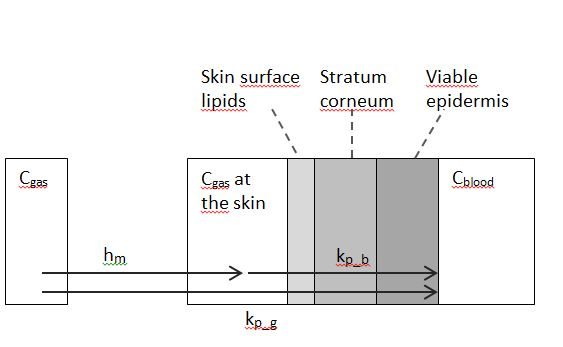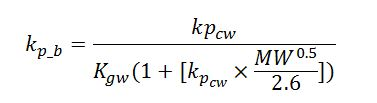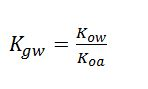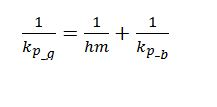## Inhalation

One of the routes of exposure to the substance, emitted from the product to the air, is inhalation. The total air concentration is the sum of the concentration of the substance in the gas phase (Cair) and the air concentration of the substance bound to airborne particles (Cair,p). From the total air concentration the daily-inhaled amount Ainh is estimated aswhere Qinh is the inhalation rate (per hour) of the exposed person, fabs,inhal is the fraction of the inhaled amount which is absorbed, and Texp is the daily exposure duration (hours per day). In this equation the concentrations Cair and Cair,p are assumed to be essentially constant over the day of exposure, which is reasonable for SVOCs.

## Dermal exposure from air

A second route of exposure to the substance from air is the dermal exposure from the air. Weschler and Nazaroff (2012) propose a method to estimate the dermal uptake from substances in the gas phase of indoor air. Following this approach, the amount absorbed through the skin, Adermal is estimated from the transdermal flux J (mass/surface area/time) asHere, Sskin is the surface area of the exposed skin.

The transdermal flux is estimated usingWhere kp_g is the trans-dermal skin permeability of the substance that describes mass transfer from the bulk indoor air into the epidermal capillaries.

Estimating kp_g

In absence of measured values of the kp_g , the DustEx tool provides a method to estimate it from basic substance properties. This method is described in detail in Weschler and Nazaroff (2012). The method assumes that the kp_g is determined by the mass transfer of the substance from air to the skin, hm and the permeability of the skin for a substance from air kp_b , which together act as a system of resistances in series.The trans-dermal skin permeability kp_g is modelled as a system of two transfer coefficients in series: the mass transfer coefficient of the susbtance from air to skin hm and the permeability of the stratum corneum/epidermis composite kp_b.

kp_b describes the permeability of the the stratum corneum/epidermis composite.

It is modelled asHere, Kgw is the gas-water partition coefficient of the substance.  It  follows from the molecular properties Kow and Koa askpcw is the permeability of the stratum corneum for a substance dissolved in water. DustEx models the kpcwusing the model by Mitragotri (2002)This equation takes the molecular weight in g/mol and predicts a kpcw in cm/s.

Finally, the total transdermal permeability coefficient kp_g is determined by approaching the skin-air system as  two resistors in series, with a resistance of  hm and Kp_b respectively## Ingestion of house dust

Exposure to substances contained in house dust is assumed to arise from the ingestion of dust. The daily intake of a substance with dust Adust is determined byWhere Adust_ingest is the amount of dust daily ingested and a fraction  fabs,oral  is actually absorbed.

In the DustEx model dermal exposure to house dust is assumed to be negligible.